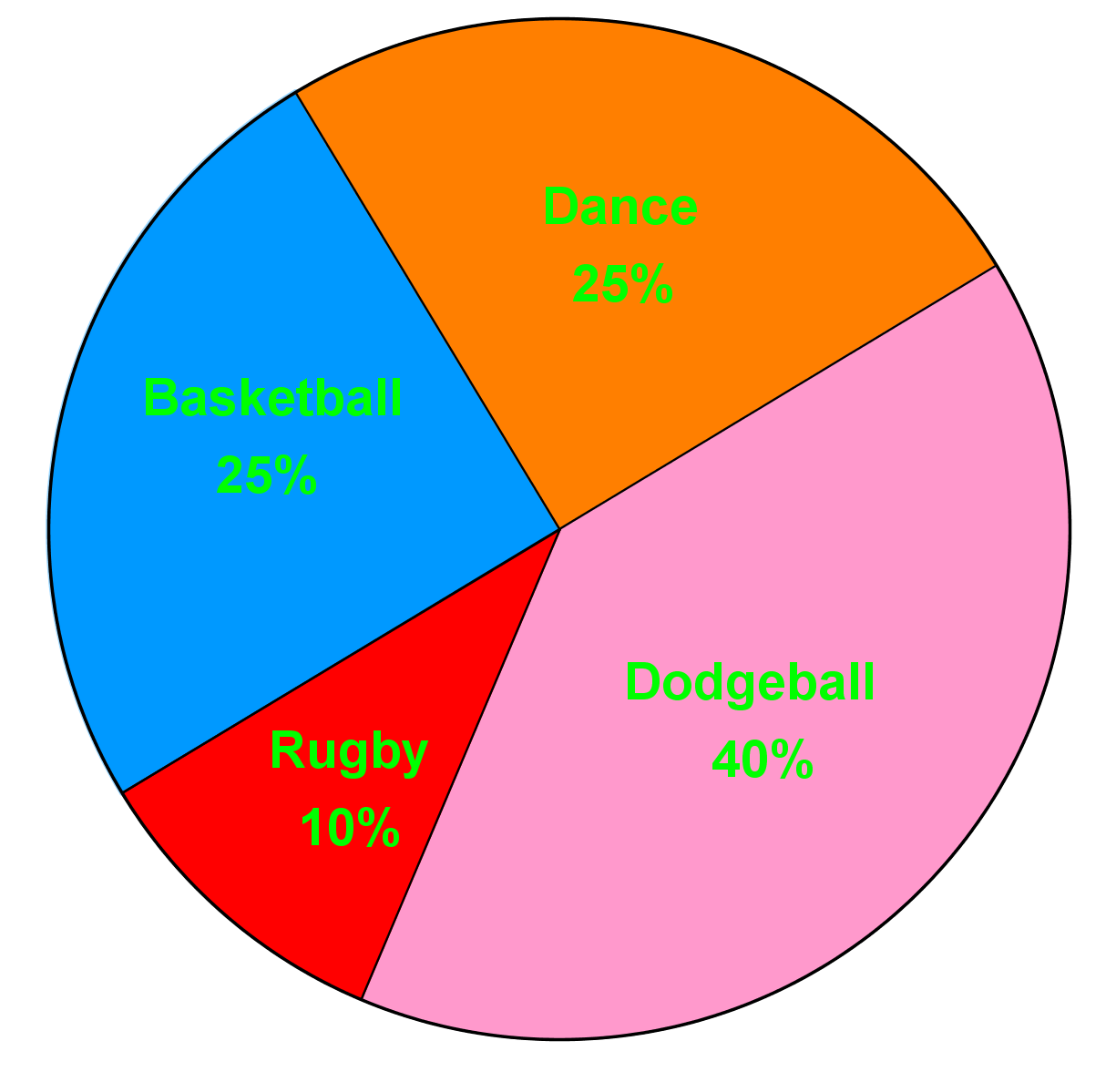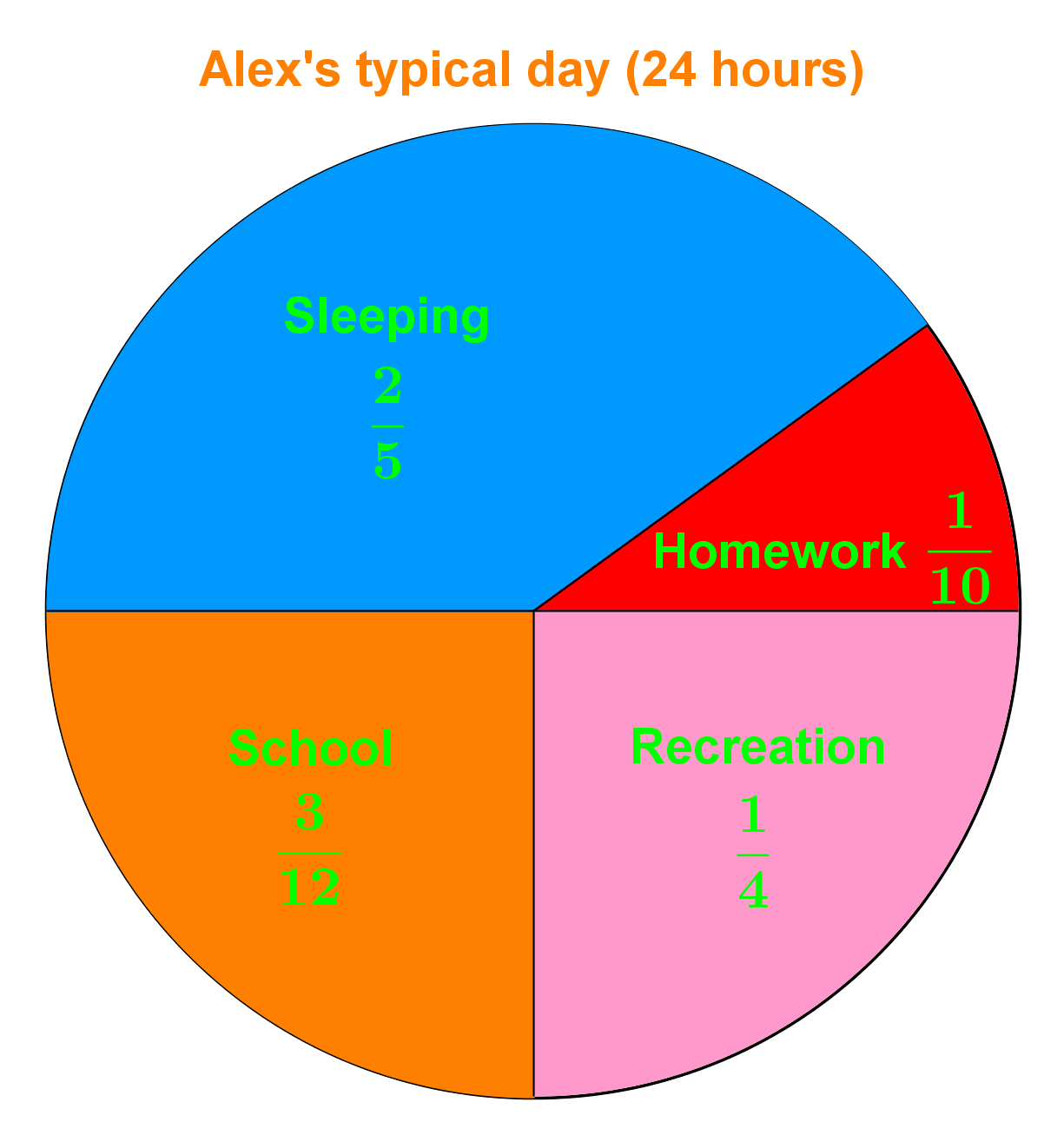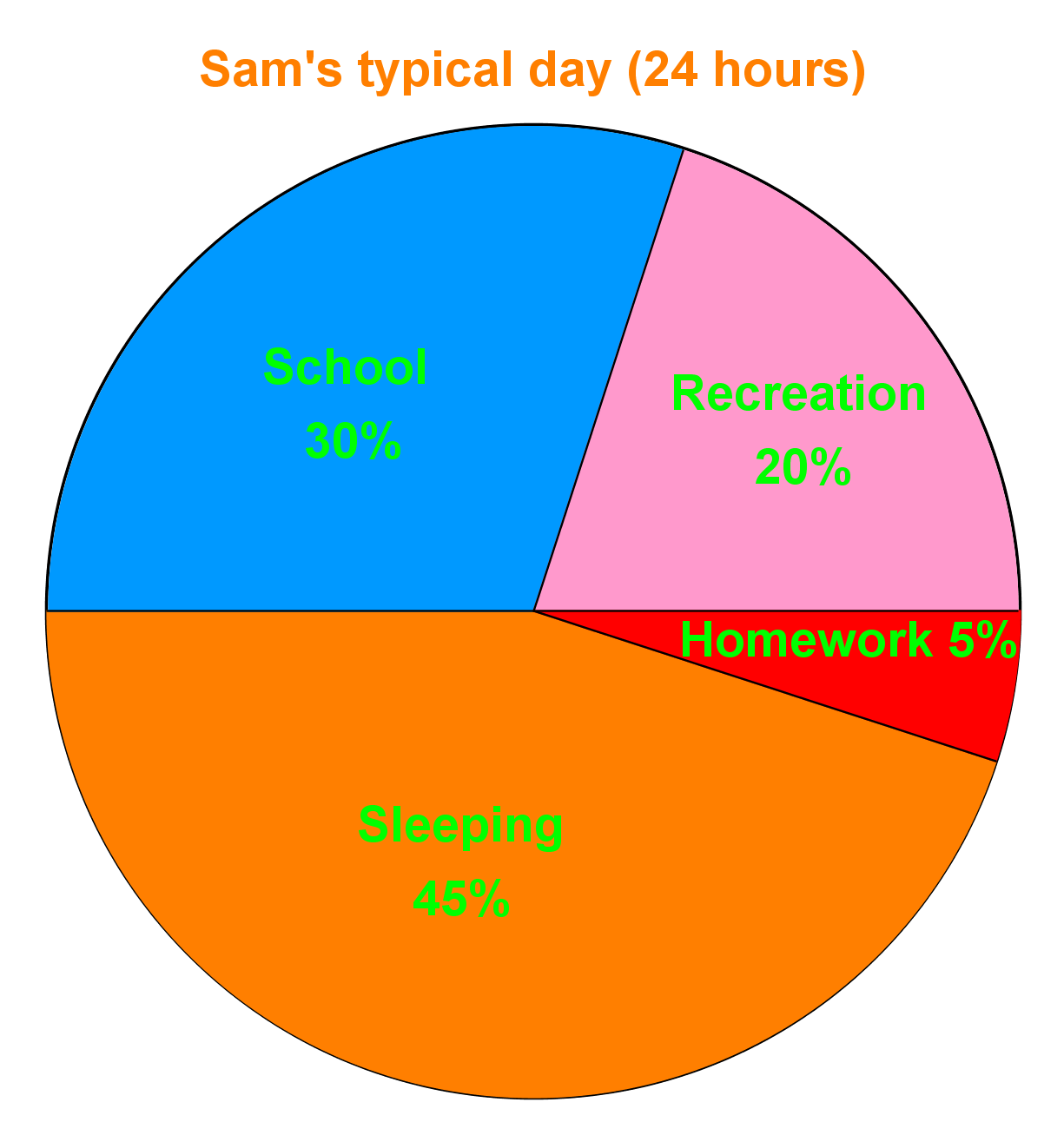# Circle graphs

### Circle graphs

Circle graphs are also known as pie charts. In this section, we are asked to interpret and create circle graphs. When interpreting the graphs, we are asked to determine the number of people represented by given percentages in our graphs. Also, we are asked to interpret tables of data and fill in these tables by converting between fractions, percentages and decimal numbers. Finally, we are asked to draw circle graphs to display data given in these tables.

#### Lessons

• Introduction
a)

b)
How to make a circle graph?

• 1.
The circle graph shows the types of activities that 200 students participated in during the school year.a)
What is the least popular activity?

b)
How many students participated in dancing?

c)
How many students do not participate in dodgeball?

d)
How many more students play basketball than rugby?

• 2.
Compare the data in these two circle graphs.a)
Who spends more time sleeping in a typical day?

b)
How much longer does Alex spend on recreation activities than Sam in a typical day?

c)
Which activity do both Alex and Sam spend most of their time on?

d)
What is the difference between the measures of the central angles in the Recreation section of Alex and Sam's day?

• 3.
30 grade 7 students were surveyed to determine their favorite type of pop.
a)
Draw a circle graph to display the data.
 Type of pop Number of students Percent of total Fractions Coke 12 Pepsi 9 7 Up 3 Ginger ale 6

• 4.
Could you use a circle graph to represent the following? Explain.
a)
The population of London each year since 1990.